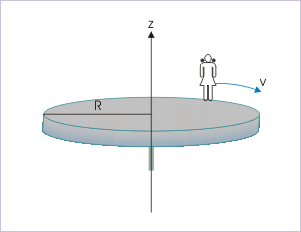# 20.6 Conservation of angular momentum (application)  (Page 3/6)

 Page 3 / 6The girl begins to walk along the rim at a speed of 1 m/s relative to the ground in clockwise direction.

Solution : Here, mass is not distributed symmetrically about the axle. The weight of girl constitutes a torque perpendicular to the axis of rotation about the point at which axle is fixed on the ground. However, torque on axle by the ground prevents rotation due to the torque resulting from girl’s weight in vertical plane. Thus, there is no external torque on the system and, therefore, angular momentum of the system of "girl and disk-axle" is conserved.The orque due to the weight of girl is balanced by restoring force of ground on the axle.

Applying law of conservation of angular momentum in vertical direction (axis of rotation), we have :

$\begin{array}{l}{L}_{i}={L}_{f}\end{array}$

Both platform and the girl are initially stationary with respect to ground. As such, initial angular momentum of the system is zero. From conservation law, it follows that the final angular momentum of the system is also zero.

But final angular momentum has two constituents : (i) final angular momentum of the platform and (ii) final angular momentum of the girl. Sum of the two angular momentums should, therefore, be zero. Let “P” and “G” subscripts denote platform and girl respectively, then :

$\begin{array}{l}{L}_{f}={L}_{\mathrm{Pf}}+{L}_{\mathrm{Gf}}=0\end{array}$

Now, MI of the circular platform about the axis of rotation is :

$\begin{array}{l}{I}_{P}=\frac{M{R}^{2}}{2}=\frac{\mathrm{100}x{3}^{2}}{2}=\mathrm{450}\phantom{\rule{2pt}{0ex}}\mathrm{kg}-{m}^{2}\end{array}$

The mass of the particles constituting the girl are at equal perpendicular distances from the axis of rotation. Its MI about the axis of rotation is :

$\begin{array}{l}{I}_{G}=m{R}^{2}=\mathrm{60}x{3}^{2}=\mathrm{540}\phantom{\rule{2pt}{0ex}}\mathrm{kg}-{m}^{2}\end{array}$

From the question, it is given that final speed of the girl around the rim of the platform is 1 m/s in clockwise direction. It means that girl has linear tangential velocity of 1 m/s with respect to ground. Her angular velocity, therefore, is :

$\begin{array}{l}{\omega }_{\mathrm{Gf}}=-\frac{v}{R}=-\frac{1}{3}\phantom{\rule{2pt}{0ex}}\mathrm{rad}/s\end{array}$

Negative sign indicates that the girl is rotating clockwise. Now, putting these values, we have :

$\begin{array}{l}{L}_{\mathrm{Pf}}+{L}_{\mathrm{Gf}}={I}_{P}{\omega }_{\mathrm{Pf}}+{I}_{G}{\omega }_{\mathrm{Gf}}=0\end{array}$

$\begin{array}{l}{\omega }_{\mathrm{Pf}}=-\frac{-1\phantom{\rule{2pt}{0ex}}x\phantom{\rule{2pt}{0ex}}\mathrm{540}}{3\phantom{\rule{2pt}{0ex}}x\phantom{\rule{2pt}{0ex}}\mathrm{450}}=\mathrm{0.4}\phantom{\rule{2pt}{0ex}}\mathrm{rad}/s\end{array}$

The positive value of final angular velocity of platform means that the platform rotates in anti-clockwise direction.

Note : Alternatively, we can find angular momentum of the girl, using the defining equation for angular momentum of a point (considering girl to be a particle like mass) as :

$\begin{array}{l}{L}_{\mathrm{Gf}}=-mv{r}_{\perp }=-\mathrm{60}x1x3=-\mathrm{180}\phantom{\rule{2pt}{0ex}}\mathrm{kg}-\frac{m}{{s}^{2}}\end{array}$

Example 3

Problem : A thin rod is placed coaxially within a thin hollow tube, which lies on a smooth horizontal table. The rod, having same mass “M” and length “L” as that of tube, is free to move within the tube. The system of "tube and rod" is given an initial angular velocity, "ω", about a vertical axis at one of its end. Considering negligible friction between surfaces, find the angular velocity of the rod, when it just slips out of the tube.

Solution : The first question that we need to answer is “why does rod slip out of the tube?”. To understand the process, let us concentrate on the motion of the rod only. Each particle of the rod, say at the far end, tends to move straight tangentially (natural tendency). However, there is no radial force available that could bend its path toward the axis of rotation. Hence, the particle tends to keep its path in tangential direction. As such, the particles have the tendency to keep themselves away from the axis of rotation. Eventually, the rod slips away from the axis of rotation.

List the application of projectile
pls explain what is dimension of 1in length and -1 in time ,what's is there difference between them
what are scalars
show that 1w= 10^7ergs^-1
what's lamin's theorems and it's mathematics representative
if the wavelength is double,what is the frequency of the wave
What are the system of units
A stone propelled from a catapult with a speed of 50ms-1 attains a height of 100m. Calculate the time of flight, calculate the angle of projection, calculate the range attained
58asagravitasnal firce
Amar
water boil at 100 and why
what is upper limit of speed
what temperature is 0 k
Riya
0k is the lower limit of the themordynamic scale which is equalt to -273 In celcius scale
Mustapha
How MKS system is the subset of SI system?
which colour has the shortest wavelength in the white light spectrum
if x=a-b, a=5.8cm b=3.22 cm find percentage error in x
x=5.8-3.22 x=2.58

#### Get Jobilize Job Search Mobile App in your pocket Now!By OpenStaxBy Mistry BhaveshBy Miranda ReisingBy Angelica LitoBy Brooke DelaneyBy OpenStaxBy OpenStaxBy Janet ForresterBy John GabrieliBy John Gabrieli